Courses

# Rigid Body Dynamics - Collisions, Classical Mechanics, CSIR-NET Physical Sciences Physics Notes | EduRev

## Physics : Rigid Body Dynamics - Collisions, Classical Mechanics, CSIR-NET Physical Sciences Physics Notes | EduRev

The document Rigid Body Dynamics - Collisions, Classical Mechanics, CSIR-NET Physical Sciences Physics Notes | EduRev is a part of the Physics Course Physics for IIT JAM, UGC - NET, CSIR NET.
All you need of Physics at this link: Physics

The Lagrangian

In the inertial space frame we found the kinetic energy of a rotating rigid body to be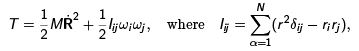M is the mass of the system and I is the inertia tensor.
The inertia tensor is a 3 × 3 matrix. Its eigenvectors are special directions within the rigid body called the principal axes. The eigenvalues of the tensor, I1 , Iand I3 , are called the principal moments of inertia.
The Lagrangian is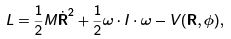where V is the external potential within which the body moves.

The angular momentum

The angular momentum L is the generalized momentum conjugate to the angular coordinates. As usual, we can write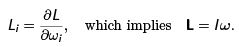In general the angular momentum is not parallel to the axis of rotation of the body.
Since I deﬁnes three independent principal axes,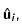, one can decompose any vector into a linear sum of components alone each of these axes. So, using the decomposition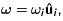, we ﬁnd that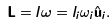In the special case when two of the ωi vanish, i.e., the angular velocity is initially in the direction of one of the principal axes, then L is parallel to the ω.

The equations of motion

In the inertial space frame, the Lagrangian is given by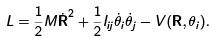So the equations of motion reduce to one set of equations for the rate of change of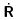and another for the rate of change of ω. We have earlier discussed why  θ do not form the components of a vector, but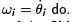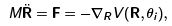where the gradient contains partial derivatives with respect to the components of R. In the absence of external forces, F = 0. The remaining three equations of motion are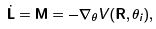where M is the external torque acting on the body.

The external torque

Representing a rigid body by N particles ﬁxed to each other,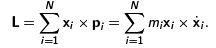Here the x are taken in an inertial frame. Then,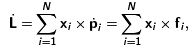where f i is the force on the i -th body. The forces of constraint satisfy f ij = −f ji . If there were only forces due to constraints, then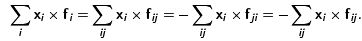As a result, this would be zero. Therefore, the net torque on the body is due to external forces only. When F = 0, the torque is called a couple.

Precession and spin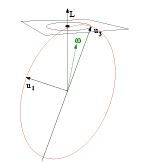For a symmetric top, I1 = I2 = I3 , the axis of symmetry is the principal axis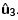. The direction of L and ω do not coincide in general. Since all directions perpendicular toare equivalent, we can choose the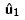directions at some time t so that L and ω are in the 13-plane. Then, since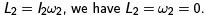Since v = ω × x, the velocity at any point in theaxis is in the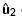direction.

This is independent of t . So, the axisrotates around the ﬁxed vector L; this is called precession. The top also rotates around; this is called spin. If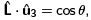, then the spin velocity is ω= L3/I3 = L cos θ/I3. The precession velocity is the component of ω1 parallel to L, so ωp = ω1 / sin θ = M /I1.

Euler angles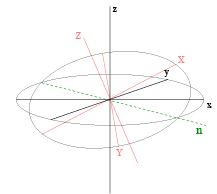Take the xyz axes to be the inertial space frame. Orient the body frame XYZ along the principal axes. If the spin velocity around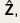,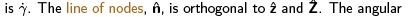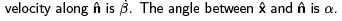Angular velocity

Problem 55: Angular velocity in Euler angles

Check that the angular velocity in the space frame, ω, is related to the rate of change of the Euler angles by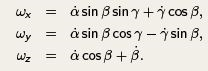Construct the rotational term in the kinetic energy using these expressions. Specialize to the cases of the spherical top and the rigid rotator and check that the correct description of free rotations is obtained in both these cases.

Simple problems

Problem 56: Precession in Euler angles For a symmetric top with I1 = I2 = I3, with the symmetry axis along, choose one of the principal axes along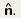. Show that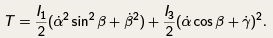Describe free rotations and ﬁnd the precession and spin angular velocities.

Problem 57: Symmetric top with a couple Take a symmetric top with I1 = I2 = I3 subject to the potential V = M cos γ . Write the Lagrangian for this problem and ﬁnd the conserved quantities and the cyclic coordinates. Using these, reduce the problem to one-dimension. Describe the general character of the motion, including nutations.

A trivial special case

In the special case when F and M are orthogonal, one can ﬁnd a spatial vector a such that

M = a × F.

Now, for any vector a(λ) = a + λF, the above equation is also satisﬁed. Additionally, if one shifts the origin of coordinates to x' = x − a, then the torque becomes M= M − a × F = 0. So, for any of these choices of coordinate systems, the external torque vanishes if in another system the force and torque are orthogonal.

Problem 58: A linear equation Given vectors F and M, one can ﬁnd a vector a such that M = a × F, because these are three equations in 3 unknowns.
Examine the linear equations in the components of a and ﬁnd what is special about them when F and M are orthogonal.

The trivial case is realistic

When the force ﬁeld is uniform, one can write each of the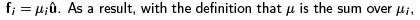the torque is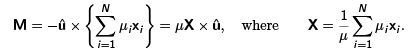In the case where the forces are purely gravitational, X = R, and the torque about the center of mass vanishes, as it does along any line in the direction of the force along the center of mass.
This is relevant to the problem discussed on September 12, and given in the mid-sem. For a satellite moving in an orbit far larger than the radius of the earth, the tidal forces may be neglected if the satellite is small. In this case the gravitational force on the satellite is exactly as above, and the motion can be analyzed as if there were no torque.

Euler equations

The simplest description of a rotating body is found in the body frame with axial directions chosen along the principal axes of the body. Clearly, for any vector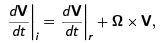where the subscript i refers to the change in the inertial system and r to the change in a system rotating with angular velocity Ω.
Using this we get Euler’s equations for rigid bodies in the body frame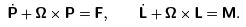Solve Euler’s equations for free rotations of a body, i.e., for M = 0.
Check that these solutions are the same as the solutions obtained earlier for free rotations, but viewed from a non-inertial frame.

Keywords

Keywords angular momentum, principal axes, space frame, torque, couple, free rotations, spherical top, rotator, symmetric top, precession, spin, Euler angles, line of nodes, nutations, Euler’s equations

Offer running on EduRev: Apply code STAYHOME200 to get INR 200 off on our premium plan EduRev Infinity!

159 docs

,

,

,

,

,

,

,

,

,

,

,

,

,

,

,

,

,

,

,

,

,

,

,

,

,

,

,

;